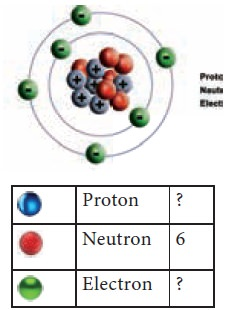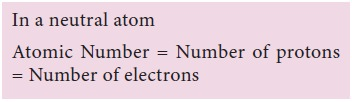Home | | Science 9th std | Discovery of Neutrons

# Discovery of Neutrons

In 1932 James Chadwick observed when Beryllium was exposed to alpha particles, particles with about the same mass as protons were emitted.

Discovery of Neutrons

In 1932 James Chadwick observed when Beryllium was exposed to alpha particles, particles with about the same mass as protons were emitted.These emitted particles carried no electrical charges. They were called as neutrons. Neutrons present in the nuclei of all the atoms except of hydrogen. The mass of a neutron is almost equal to the mass of proton. Neutron is represented by n.

## 1. Composition of nucleus

Electrons have a negligible mass; hence the mass of the atom mainly depends on the mass of the nucleus. Nucleus of an atom consists of two components, they are protons and neutrons.

Protons are positively charged. Protons repel each other because of their like charges. Hence more than one proton cannot be packed in a small volume to form a stable nucleus, unless neutrons are present.

Neutrons reduce the repulsive force between the positively charged protons and contribute to the force that holds the particles in the nucleus together.## 2. Nucleons

The elementary particles such as protons and neutrons are collectively called as Nucleons. Why are atoms neutral? Because an atom contains the same number of protons and electrons and hence itâ€™s neutral.

### Characteristics of fundamental particles

The physical and chemical properties of elements and their compounds can be explained by the fundamental particles of an atom. The fundamental particles are proton, neutron and electron.### Terminology

#### Atomic Number (Z)

The  figure shown here represents an atom.

Using the colour code given belowCount the number of protons, electrons and complete the table. Are the number of protons and electrons the same?

An atom of an element has its own characteristic number of protons in its nucleus, which distinguishes it from the atoms of other elements. Hence proton is considered to be the nger print of an atom.

This characteristic number (Number of protons) is called the atomic number of the element. Atomic number is denoted by Z.

What is the Atomic number of the above element?

Since there are 6 protons, the atomic number = 6.

The number of electrons = 6, which is the same as the atomic number.

Atomic number of an atom is therefore equal to the number of protons and it is also equal to the number of electrons present.Illustration:

An atom has 11 protons, 11 electrons and 12 neutrons. What is the atomic number and the name of the element?

Atomic number = Number of protons = Number of electrons

Number of protons = Number of electrons = 11

Atomic number = 11

Name of the element is Sodium.#### Mass Number:(A)

From Rutherfordâ€™s experiment it was clear that the mass of the atom is concentrated in the nucleus. is means that mass of an atom is practically due to protons and neutrons which are present in the nucleus. Protons and neutrons together are also called nucleons.

Mass number of the element is the total number of protons and neutrons present in the nucleus.

Mass number is denoted by AFor example if an atom has 3 protons, 3 electrons and 4 neutrons, then its mass number will be equal to 7 (3 protons+ 4 neutrons)Symbolic representation of an atom using Atomic Number and Mass Number

An atom can be represented by its symbol with atomic number as subscript and mass number as superscript.For example, nitrogen is written asHere 7 is its atomic number and 14 is its mass number.

### Relationship between Mass Number and Atomic Number:

Mass Number (A) = Atomic Number (z) + Number of Neutrons(n)

Atomic Number (Z) = Number of Protons or Number of Electrons

A = Z + n

### Sample Problem (solved) :

Calculate the atomic number of an element whose mass number is 39 and number of neutrons is 20. Also find the name of the element.

Solution:

Mass Number = Atomic Number + Number of neutrons

Atomic Number = Mass Number â€“ Number of neutrons

= 39 â€“ 20

Atomic Number = 19

Element having Atomic Number 19 is Potassium (K)

Complete the following table: Pair workStudy Material, Lecturing Notes, Assignment, Reference, Wiki description explanation, brief detail
9th Science : Atomic Structure : Discovery of Neutrons |